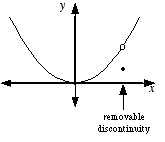index: click on a letter A B C D E F G H I J K L M N O P Q R S T U V W X Y Z A to Z index index: subject areas numbers & symbols sets, logic, proofs geometry algebra trigonometry advanced algebra & pre-calculus calculus advanced topics probability & statistics real world applications multimedia entrieswww.mathwords.com about mathwords website feedback

 Removable Discontinuity Hole A hole in a graph. That is, a discontinuity that can be "repaired" by filling in a single point. In other words, a removable discontinuity is a point at which a graph is not connected but can be made connected by filling in a single point. Formally, a removable discontinuity is one at which the limit of the function exists but does not equal the value of the function at that point; this may be because the function does not exist at that point.See also